Скачать презентацию 1 problem set 11 from Binmore s Fun

f4d2749443eabf3f0e583826ce00cdee.ppt

• Количество слайдов: 281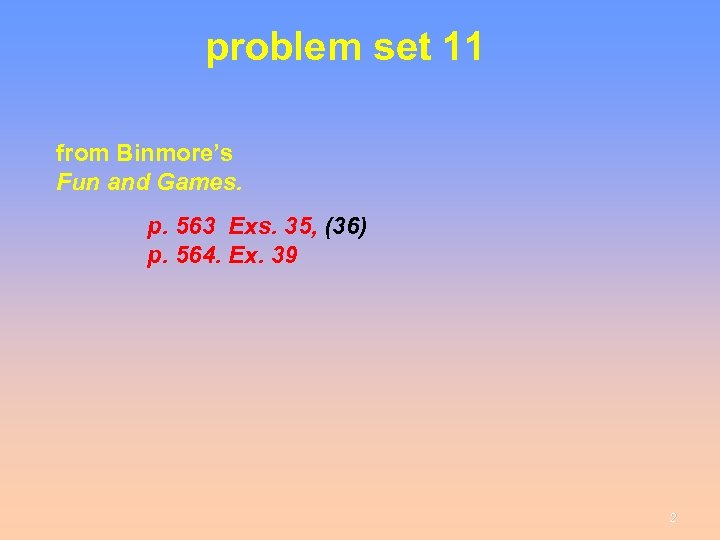problem set 11 from Binmore’s Fun and Games. p. 563 Exs. 35, (36) p. 564. Ex. 39 2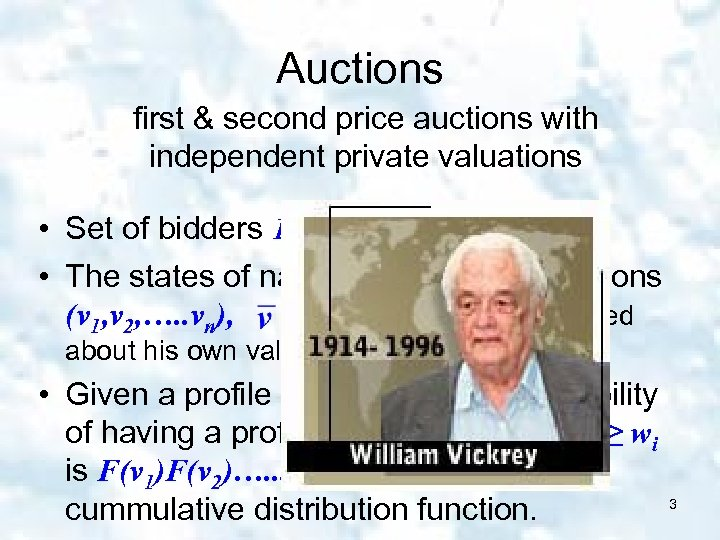Auctions first & second price auctions with independent private valuations • Set of bidders 1, 2…. n • The states of nature: Profiles of valuations (v 1, v 2, …. . vn), Each is informed about his own valuation only. • Given a profile (v 1, v 2, …. . vn), the probability of having a profile (w 1, w 2, …. . wn), s. t. vi ≥ wi is F(v 1)F(v 2)…. . . F(vn). Where F() is a cummulative distribution function. 3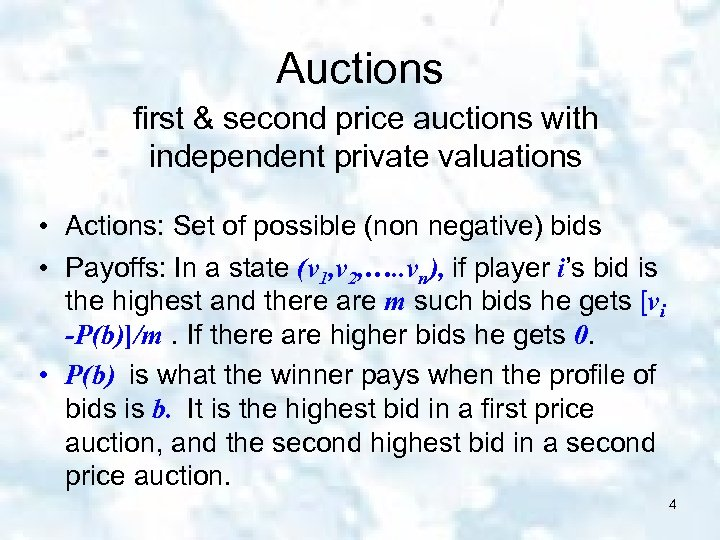Auctions first & second price auctions with independent private valuations • Actions: Set of possible (non negative) bids • Payoffs: In a state (v 1, v 2, …. . vn), if player i’s bid is the highest and there are m such bids he gets [vi -P(b)]/m. If there are higher bids he gets 0. • P(b) is what the winner pays when the profile of bids is b. It is the highest bid in a first price auction, and the second highest bid in a second price auction. 4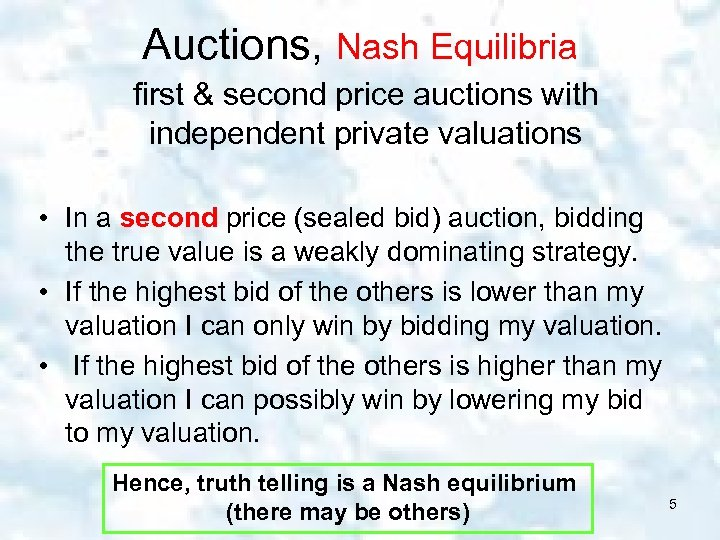Auctions, Nash Equilibria first & second price auctions with independent private valuations • In a second price (sealed bid) auction, bidding the true value is a weakly dominating strategy. • If the highest bid of the others is lower than my valuation I can only win by bidding my valuation. • If the highest bid of the others is higher than my valuation I can possibly win by lowering my bid to my valuation. Hence, truth telling is a Nash equilibrium (there may be others) 5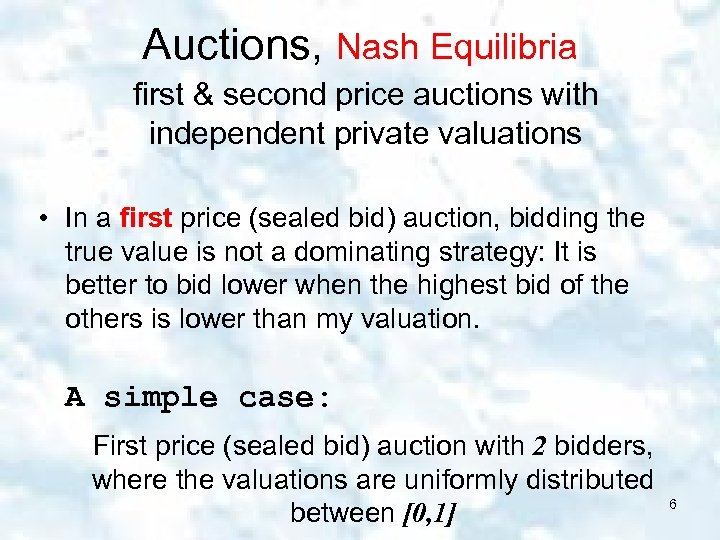Auctions, Nash Equilibria first & second price auctions with independent private valuations • In a first price (sealed bid) auction, bidding the true value is not a dominating strategy: It is better to bid lower when the highest bid of the others is lower than my valuation. A simple case: First price (sealed bid) auction with 2 bidders, where the valuations are uniformly distributed between [0, 1] 6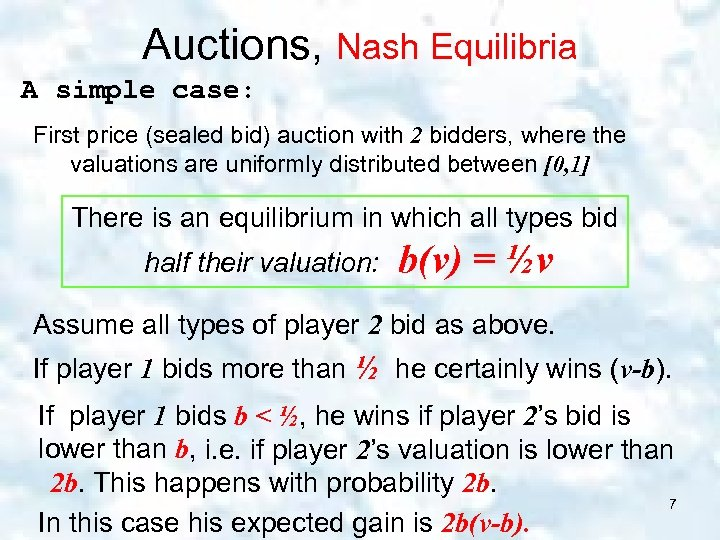Auctions, Nash Equilibria A simple case: First price (sealed bid) auction with 2 bidders, where the valuations are uniformly distributed between [0, 1] There is an equilibrium in which all types bid half their valuation: b(v) = ½v Assume all types of player 2 bid as above. If player 1 bids more than ½ he certainly wins (v-b). If player 1 bids b < ½, he wins if player 2’s bid is lower than b, i. e. if player 2’s valuation is lower than 2 b. This happens with probability 2 b. 7 In this case his expected gain is 2 b(v-b).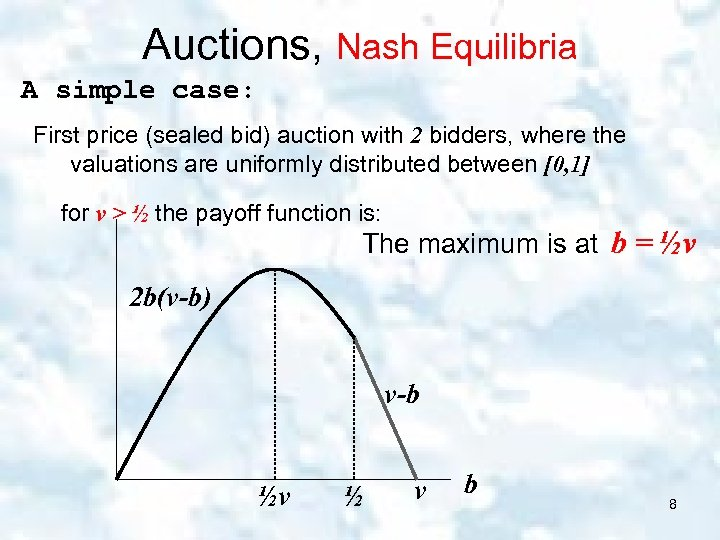Auctions, Nash Equilibria A simple case: First price (sealed bid) auction with 2 bidders, where the valuations are uniformly distributed between [0, 1] for v > ½ the payoff function is: The maximum is at b = ½v 2 b(v-b) v-b ½v ½ v b 8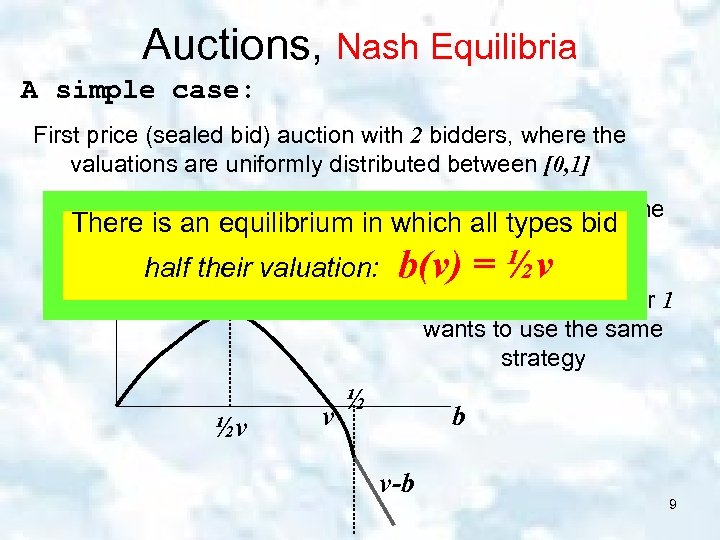Auctions, Nash Equilibria A simple case: First price (sealed bid) auction with 2 bidders, where the valuations are uniformly distributed between [0, 1] for v < ½ the payoff function is somewhat different but the There is an equilibrium in which all types bid maximum is as before at b = ½v half their valuation: 2 b(v-b) ½v v b(v) = ½v i. e. each type of player 1 wants to use the same strategy ½ b v-b 9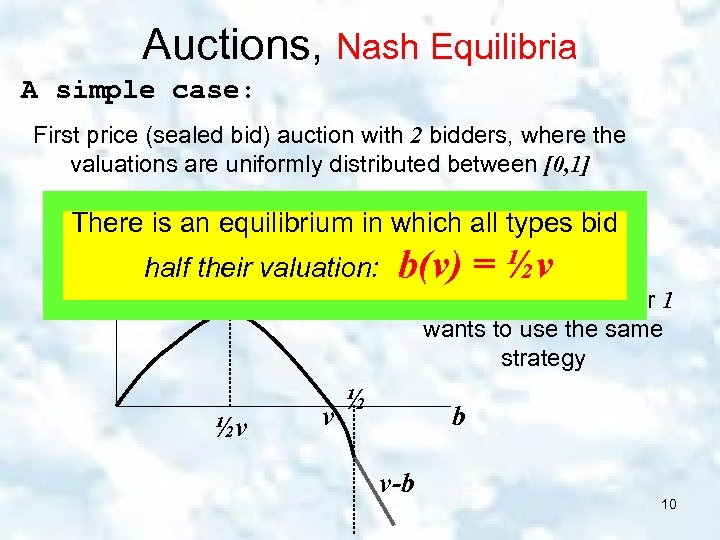Auctions, Nash Equilibria A simple case: First price (sealed bid) auction with 2 bidders, where the valuations are uniformly distributed between [0, 1] There is an equilibrium in which all types bid half their valuation: 2 b(v-b) ½v v b(v) = ½v i. e. each type of player 1 wants to use the same strategy ½ b v-b 10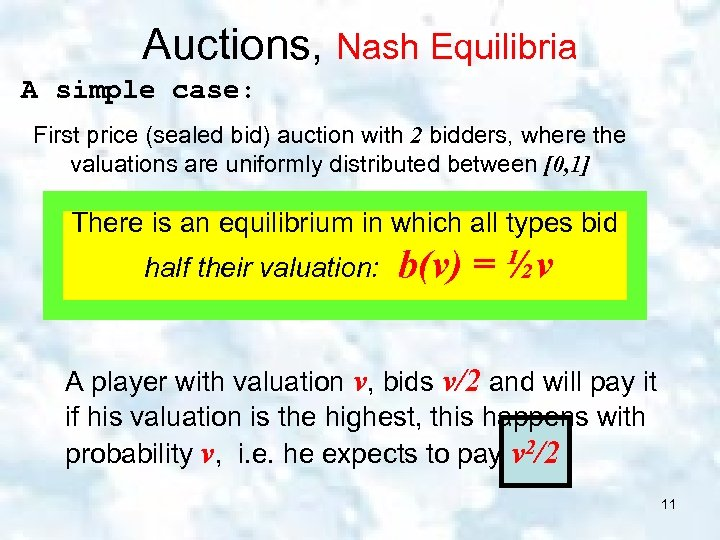Auctions, Nash Equilibria A simple case: First price (sealed bid) auction with 2 bidders, where the valuations are uniformly distributed between [0, 1] There is an equilibrium in which all types bid half their valuation: b(v) = ½v A player with valuation v, bids v/2 and will pay it if his valuation is the highest, this happens with probability v, i. e. he expects to pay v 2/2 11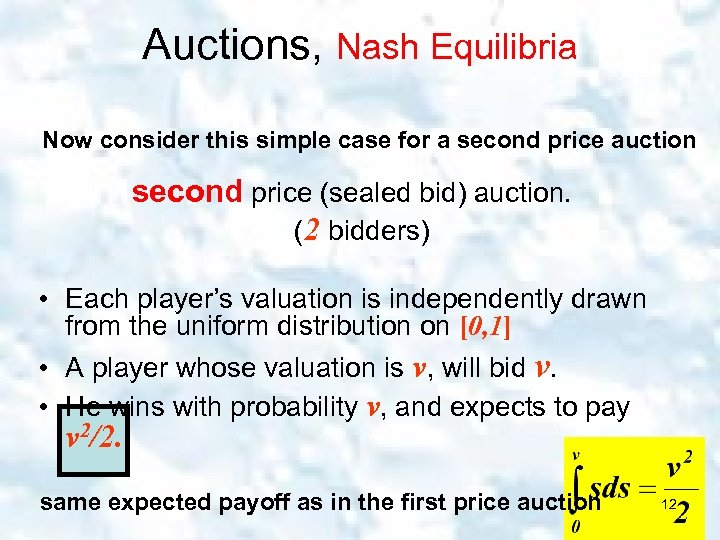Auctions, Nash Equilibria Now consider this simple case for a second price auction second price (sealed bid) auction. (2 bidders) • Each player’s valuation is independently drawn from the uniform distribution on [0, 1] • A player whose valuation is v, will bid v. • He wins with probability v, and expects to pay v 2/2. same expected payoff as in the first price auction 12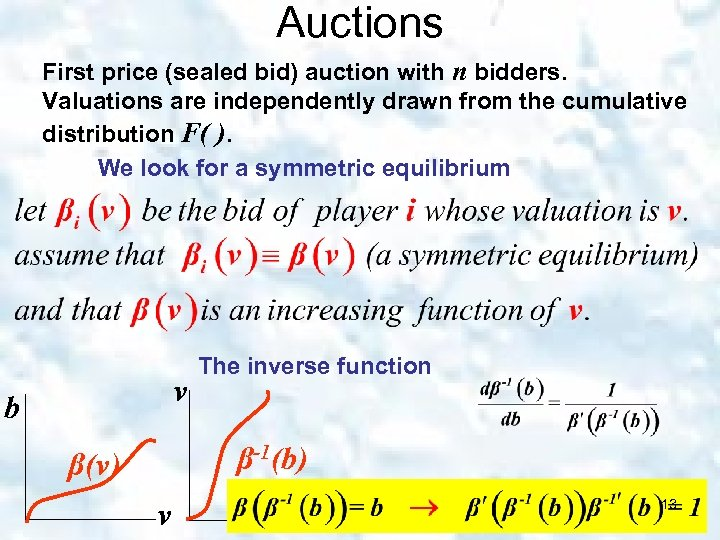Auctions First price (sealed bid) auction with n bidders. Valuations are independently drawn from the cumulative distribution F( ). We look for a symmetric equilibrium v b The inverse function β-1(b) β(v) v b 13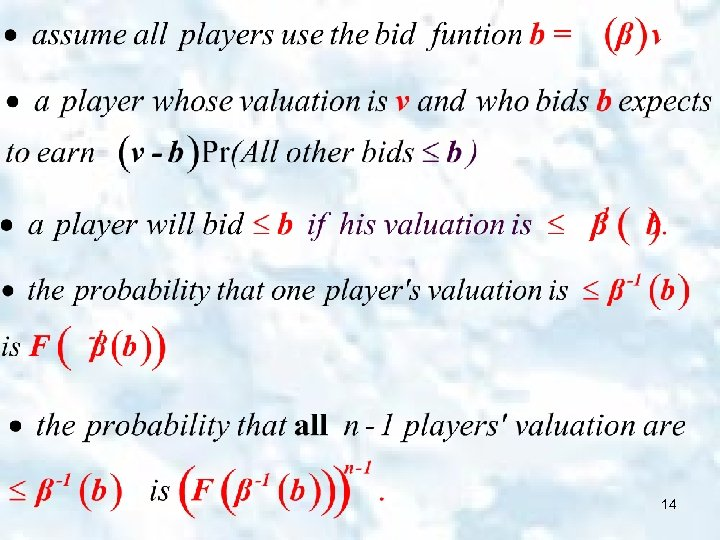1415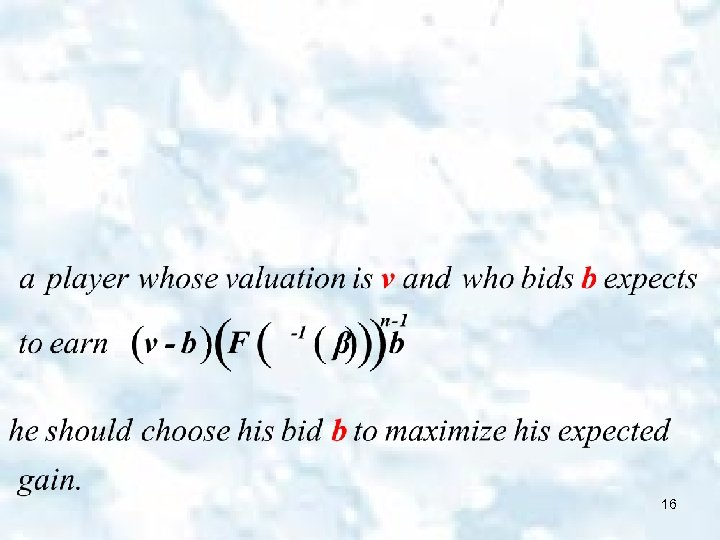16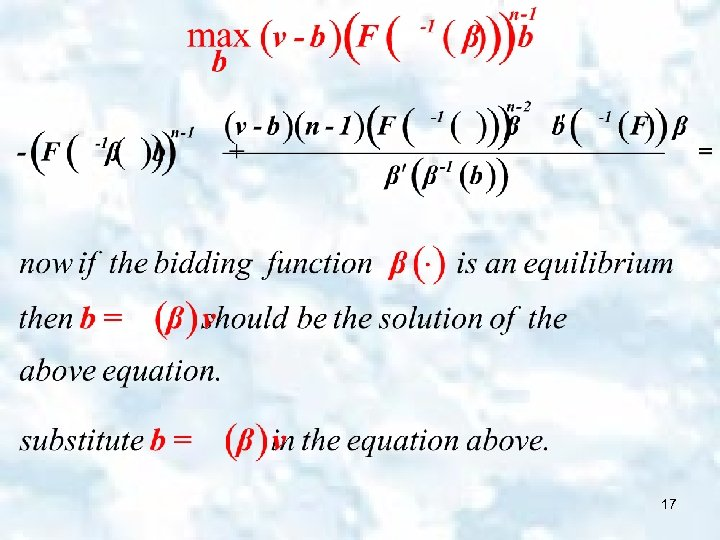1718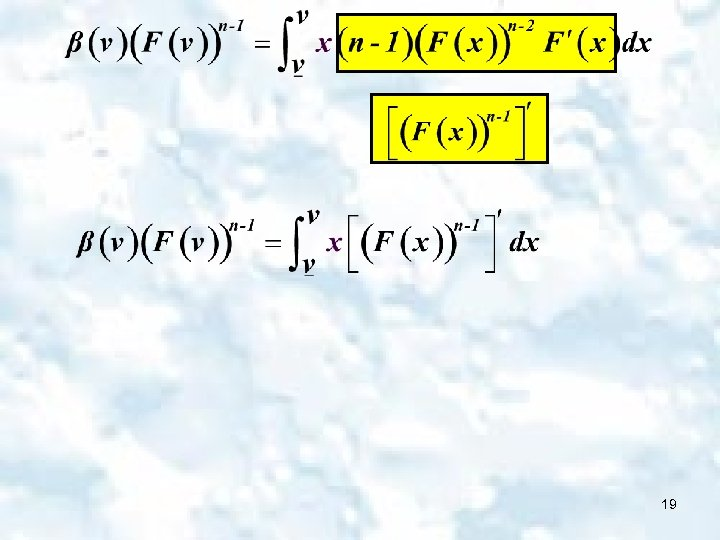19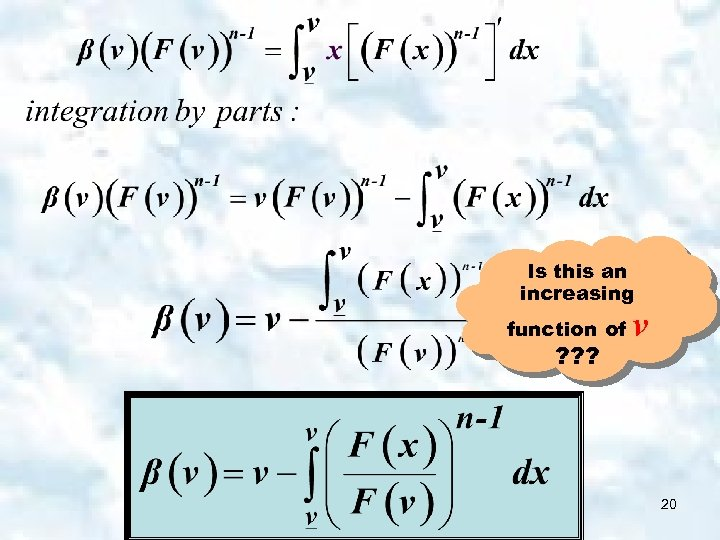Is this an increasing function of ? ? ? v 20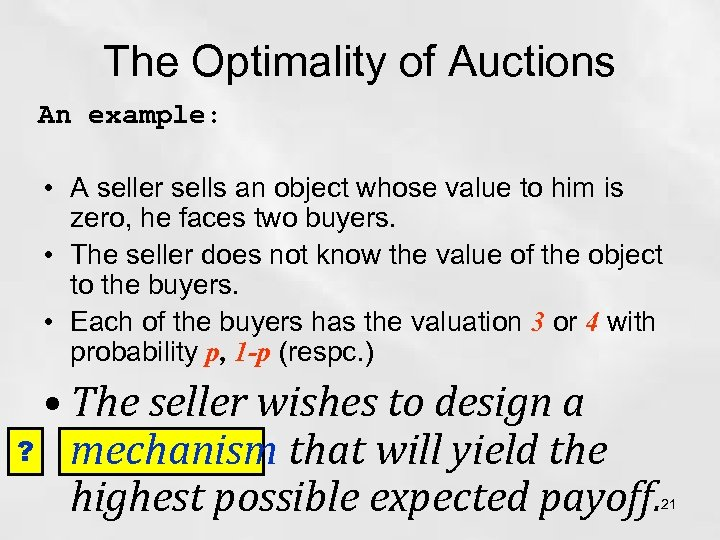The Optimality of Auctions An example: • A seller sells an object whose value to him is zero, he faces two buyers. • The seller does not know the value of the object to the buyers. • Each of the buyers has the valuation 3 or 4 with probability p, 1 -p (respc. ) • The seller wishes to design a ? mechanism that will yield the highest possible expected payoff. 21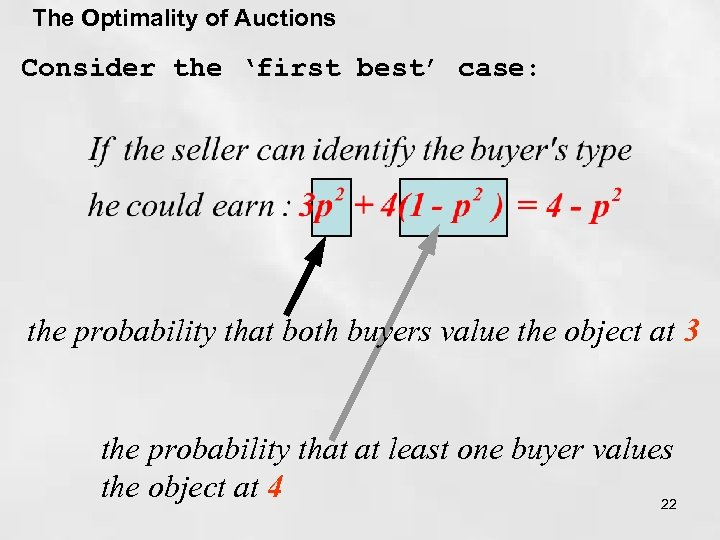The Optimality of Auctions Consider the ‘first best’ case: the probability that both buyers value the object at 3 the probability that at least one buyer values the object at 4 22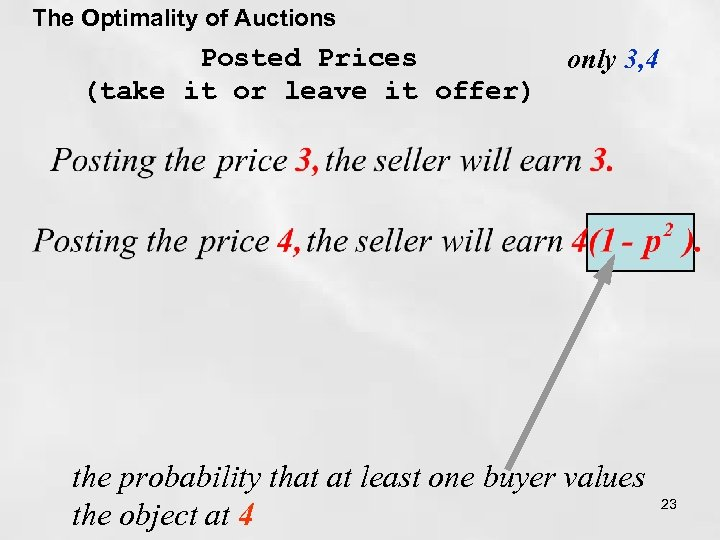The Optimality of Auctions Posted Prices (take it or leave it offer) only 3, 4 the probability that at least one buyer values the object at 4 23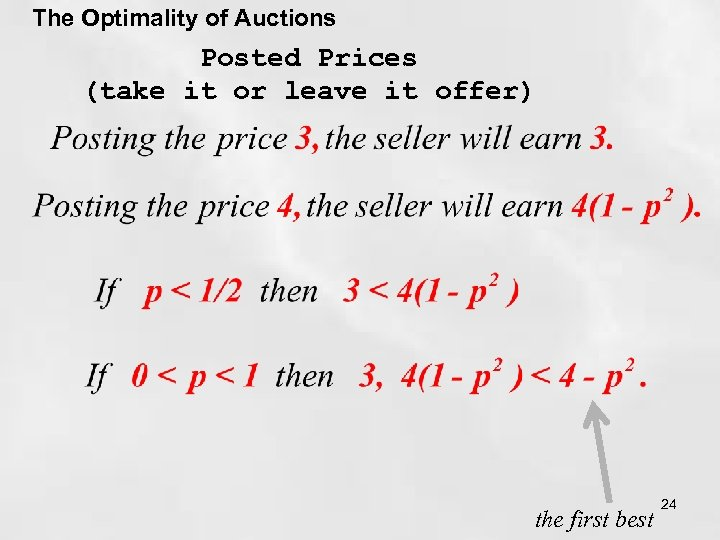The Optimality of Auctions Posted Prices (take it or leave it offer) the first best 24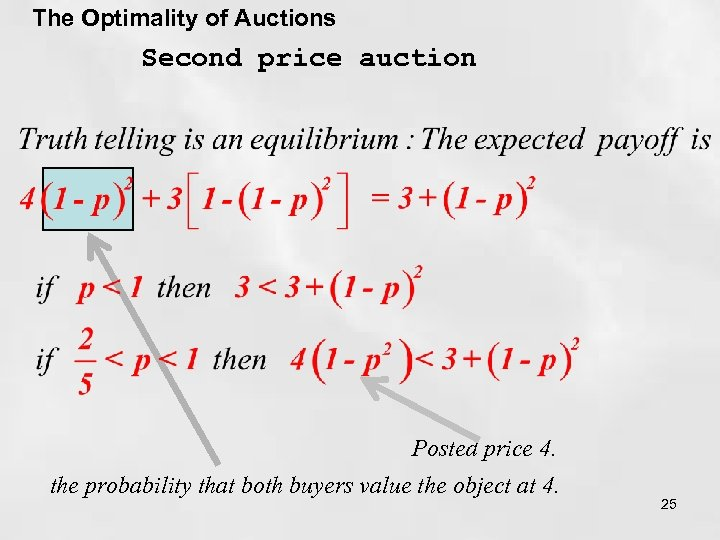The Optimality of Auctions Second price auction Posted price 4. the probability that both buyers value the object at 4. 25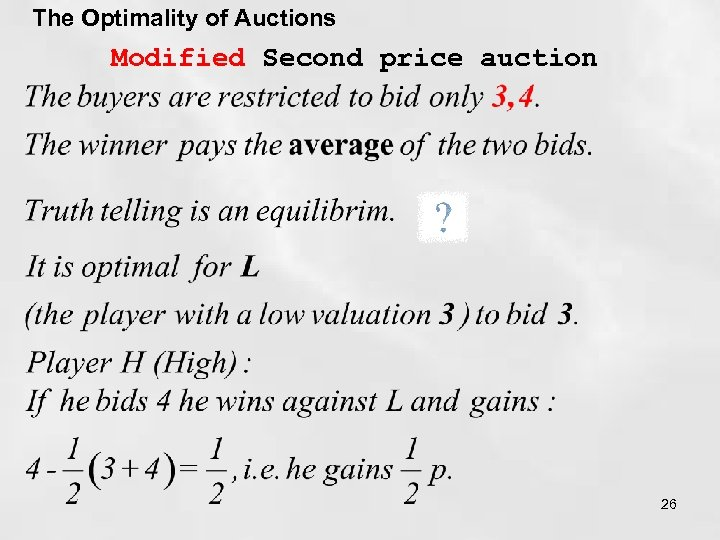The Optimality of Auctions Modified Second price auction 26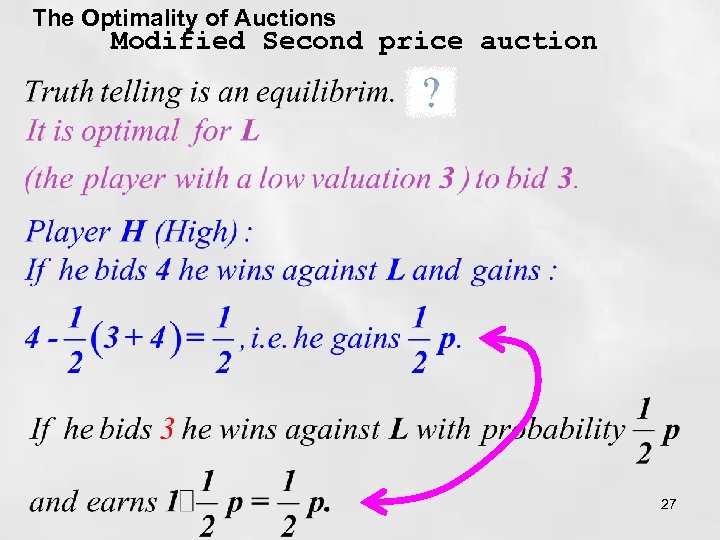The Optimality of Auctions Modified Second price auction 27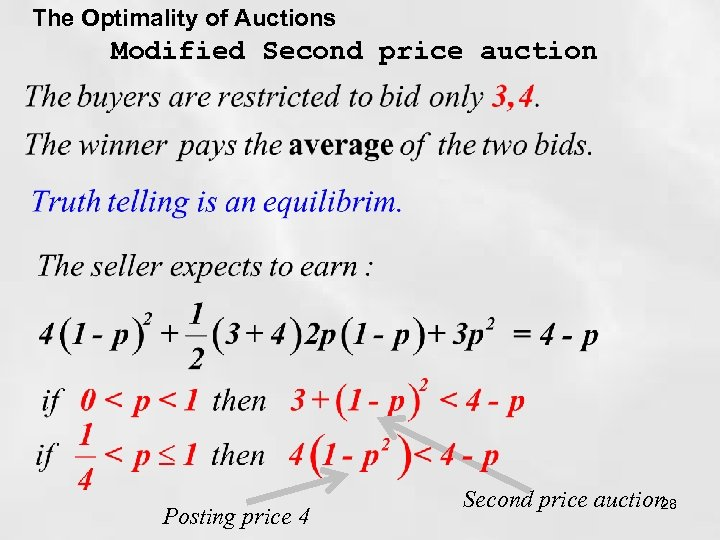The Optimality of Auctions Modified Second price auction Posting price 4 Second price auction 28